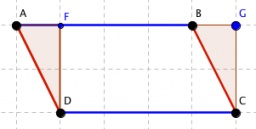# Circumference 6355

The circumference of the parallelogram is 486 cm. The length of one side is 1.7 times the length of the shorter side. How long are the sides of the parallelogram?

a =  153
b =  90

### Step-by-step explanation:

2(a+b)=486
a = 1.7 b

2•(a+b)=486
a = 1.7•b

2a+2b = 486
a-1.7b = 0

a = 153
b = 90

Our linear equations calculator calculates it.Did you find an error or inaccuracy? Feel free to write us. Thank you!

Tips for related online calculators
Do you have a linear equation or system of equations and looking for its solution? Or do you have a quadratic equation?
Do you want to convert length units?

#### Grade of the word problem:

We encourage you to watch this tutorial video on this math problem: